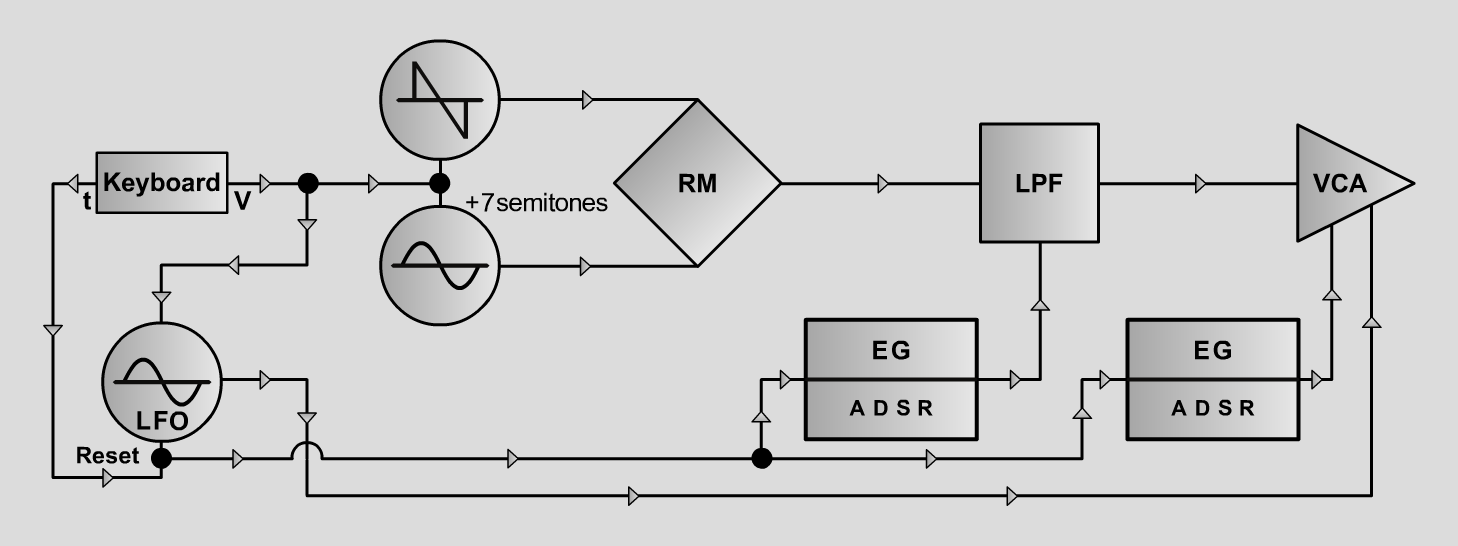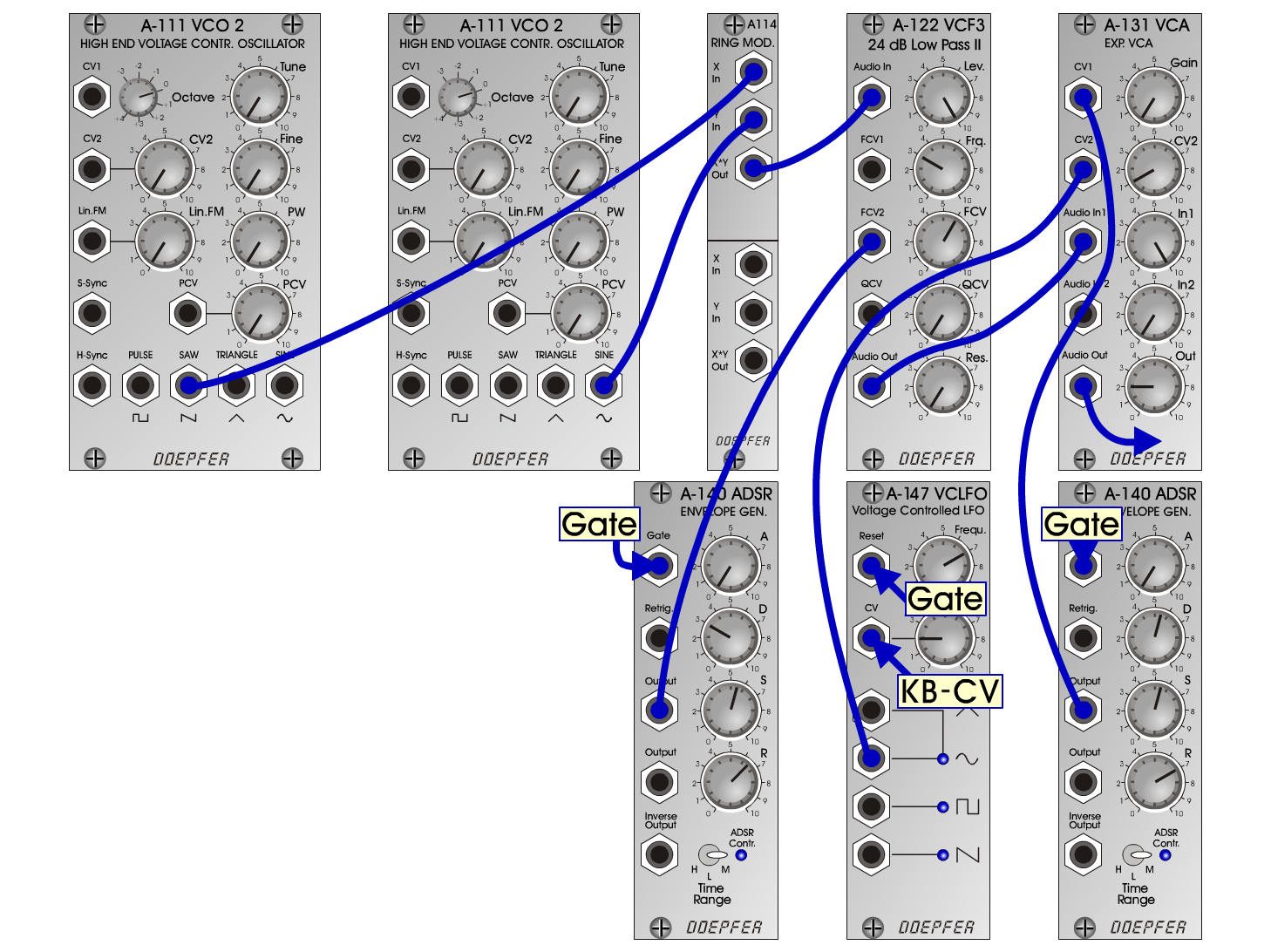B e l lInstrumentsD o e p f e r A - 100 Connections: Settings: Bell1 A-111/1 (Saw) <=> A-114 (X In)  A-111/2 (Sine) <=> A-114 (Y In)  A-114 (Out) <=> A-122 (Audio In)  A-122 (Audio Out) <=> A-131 (Audio In)  A-140/1 (Output) <=> A-122 (FCV2)  A-140/2 (Output) <=> A-131 (CV1)  A-147 (Sine) <=> A-131 (CV2)  KB-CV <=> A-147 (CV)  KB-Gate <=> A-147 (Reset) A-122 (Level = 10, Freq = 3, FCV = 6, Res = 0)  A-140/1 (A = 0, D = 3, S = 5.5, R = 6.5, Range = M)  A-140/2 (A = 0, D = 5.5, S = 5.5, R = 7, Range = M)  A-147 (Freq = 7, CV = 2)  A-131 (Gain = 0, CV2 = 1, Audio In 1 = 10, Audio Out = 2) Bell2 A-122 (Level = 10, Freq = 5, FCV = 2, Res = 0)  A-140/1 (A = 0, D = 3, S = 5.5, R = 6.5, Range = M)  A-140/2 (A = 0, D = 5.5, S = 5.5, R = 7, Range = M)  A-147 (Freq = 7, CV = 2)  A-131 (Gain = 0, CV2 = 1, Audio In 1 = 10, Audio Out = 2) Notes: Tune A-111/2  7 semitones higher than A-111/1. Josef MuellerSound samples Bach Inventio 6 (BWV 777) Bell 1 A Bell 1 B Bell 1 C Bell 2 A Bell 2 B Bell 2 C
 Bell1-A.mp3   Bell1-B   Bell1-C MP3 Bell2-A.mp3   Bell2-B   Bell2-C MP3 Bach Inventio 6.mp3 MP3  (BWV 777)